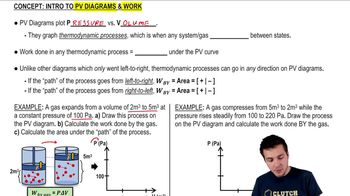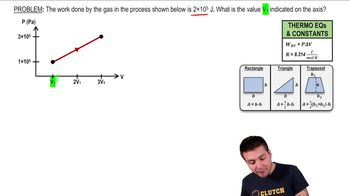Start typing, then use the up and down arrows to select an option from the list.
5:53 minutes
Problem 19c
Textbook Question

# A cylinder contains 0.0100 mol of helium at T = 27.0°C. (a) How much heat is needed to raise the temperature to 67.0°C while keeping the volume constant? Draw a pV-diagram for this process. (b) If instead the pressure of the helium is kept constant, how much heat is needed to raise the temperature from 27.0°C to 67.0°C? Draw a pV-diagram for this process. (c) What accounts for the difference between your answers to parts (a) and (b)? In which case is more heat required? What becomes of the additional heat? (d) If the gas is ideal, what is the change in its internal energy in part (a)? In part (b)? How do the two answers compare? Why?Verified Solution
This video solution was recommended by our tutors as helpful for the problem above.
29views